Next: Kepler's laws Up: Keplerian orbits Previous: Keplerian orbits

# Introduction

Newtonian mechanics was initially developed in order to account for the motion of the planets around the Sun. Let us now examine this problem. Suppose that the Sun, whose mass is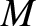, is located at the origin of our coordinate system. Consider the motion of a general planet, of mass, that is located at position vector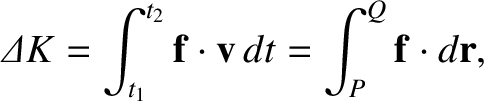. Given that the Sun and all of the planets are approximately spherical, the gravitational force exerted on our planet by the Sun can be written (see Chapter 3)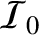(4.1)

An equal and opposite force to that given in Equation (4.1) acts on the Sun. However, in this chapter, we shall assume that the Sun is so much more massive than the planet that this force does not cause the Sun's position to shift appreciably. Hence, the Sun will always remain at the origin of our coordinate system. Likewise, we shall neglect the gravitational forces exerted on our chosen planet by the other bodies in the solar system, compared to the gravitational force exerted on it by the Sun. This is reasonable because the Sun is much more massive (by a factor of, at least,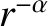) than any other solar system body. Thus, according to Equation (4.1) and Newton's second law of motion, the equation of motion of our planet (which can effectively be treated as a point object--see Chapter 3) takes the form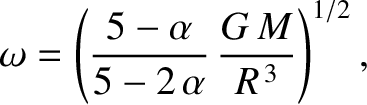(4.2)

Note that the planet's mass,, has canceled out on both sides of this equation.Next: Kepler's laws Up: Keplerian orbits Previous: Keplerian orbits
Richard Fitzpatrick 2016-03-31